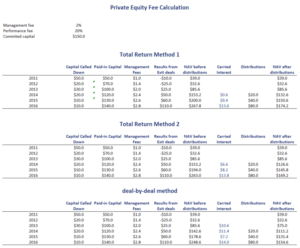# Distribution Waterfall

A distribution waterfall in private equity dictates when carried interest is paid to the general partner. While this may sound trivial, there are in fact at least three different methods to determine performance on which carried interest can be calculated.

On this page, we discuss simple versions of the three most commonly used methods that are used in practice to determine carried interest. At the bottom of the page, we provide a private equity distribution waterfall model in Excel. The spreadsheet illustrates the different methods we discuss in more detail below.

## Distribution waterfall model definition

A private equity waterfall model is typically put in place to make sure the the general partner (GP) does not the receive carried interest “too early”. That is, a distribution waterfall is a method to ensure that the manager only receives a performance fee after the limited partners (LPs) have made a return on investment. Similar method are commonly used in for hedge funds (high-water mark) and real estate as well.

## Distribution waterfall types

We can distinguish at least three types. Many more ways are possible, but we discuss the three most commonly used types in private equity and real estate.

The three methods to calculate carried interest are:

1. Deal-by-deal method: In this case, the carried interest is calculated separately after each individual deal. For example, suppose a deal generates a return of \$10 million, in that case 20% of the gain goes to the GPs.
2. Total return method 1: Under the first total return method, the carried interest is calculated on the full portfolio after committed capital is returns
3. Total return method 2: under the second total return method, carried interest is paid only when the value of the portfolio exceeds invested capital by some minimum amount (e.g. 20%).

Notice that under the total return methods, the difference is that the first method uses committed capital, whereas the second method uses capital actually invested.

## Distribution waterfall example

Let’s turn to an example. In the following table, we apply the three methods to calculate carried interest. The distribution waterfall model Excel we used to create the table can be found below.## Summary

We discussed the private equity distribution waterfall. This corporate governance term of a private equity firm determines how carried interest is calculated. Thus, it is a very important clause that investors should understand well. A complicating factor investors should consider is that different calculation methods exist.